Jet Set Go! All about Aeroplanes Jet Set Go! All about Aeroplanes

# Division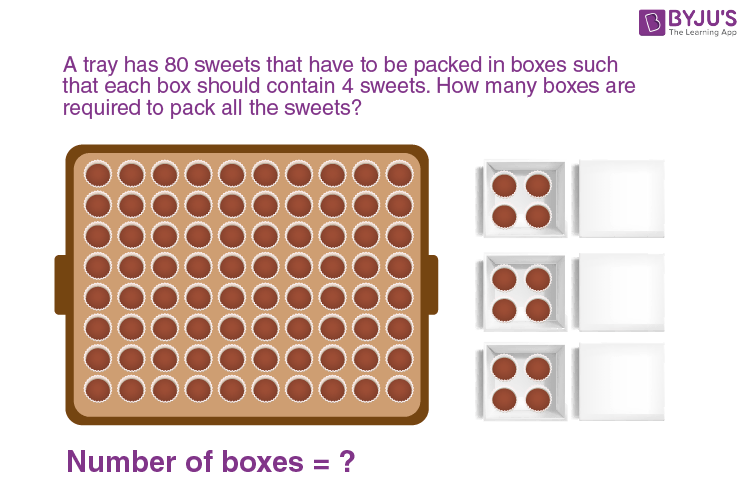Let’s consider the example given in the above figure. This method is actually about how children divide when they distribute some objects repeatedly. In this case, they might first pack 4 sweets each in 10 or 15 boxes and then next pack the remaining sweets in the second round to simplify the procedure. They could as well pack by taking a few more boxes. Children can, thus, use any way to complete the process of division. This is the beauty of this method.

In this article, you will the mathematical definition of division, rules of division, examples of division in different situations along with practice questions.

## What is Division?

The division is the process of sharing a collection of items into equal parts and is one of the basic arithmetic operations in maths. We may face different situations every day where we use the division technique.

Division by Zero:

The division of any number by zero is undefined. In this case, the result can be written as infinity. For example, 15/0 = ∞, i.e. undefined.

Division Algorithm:

Division of any expression follows the division algorithm given below.

Dividend = Divisor × Quotient + Remainder

This algorithm helps in finding the missing numbers in the division problem.## Division Rule

Division rule involves four steps; they are:

Step 1: Identify the dividend and divisor and then write in the respective places.

Step 2: Multiply the divisor with a suitable number such that we get a result close to the dividend.

Step 3: Subtract the values in the dividend column.

Step 4: Now, bring down the result and repeat the preceding two steps until the remainder is less than the divisor.

This can be understood clearly with the help of the below figure: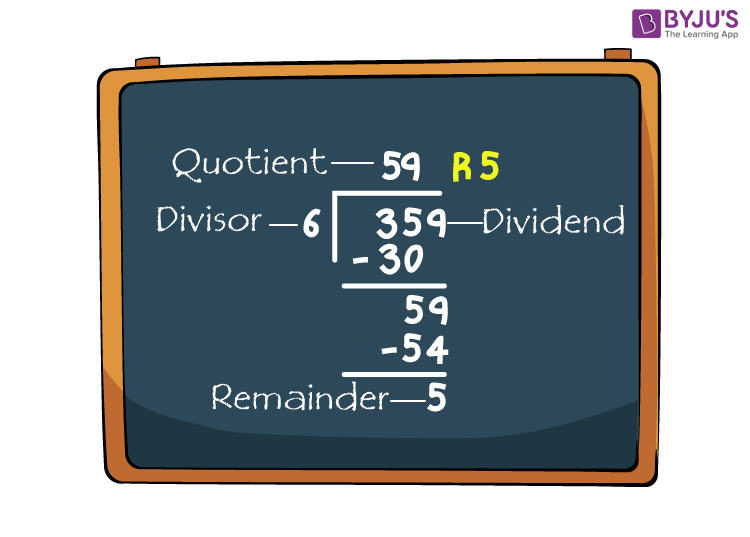## Division Symbol

We can represent the division with ÷, slash (/) or a horizontal line ( _ ). We can use these symbols at our convenience when dealing with different types of computations or division questions. Here, x/y or

$$\begin{array}{l}\frac{x}{y}\end{array}$$
can be read as “x by y” or “x over y”.

For example, the division of 48 by 6 can be expressed in either of the following ways.

48 ÷ 6 = 8

48/6 = 8

Or

$$\begin{array}{l}\frac{48}{6}=8\end{array}$$

However, the result is the same in all three representations.

Verification of Division Result:

It is easy to verify whether the obtained answer is accurate or incorrect in nature. The reverse process of multiplication is termed as division. The method to verify the answer using the given information is discussed below. Consider a simple example of 6 being divided by 2 gives 3. It can be mathematically written as

6 ÷ 2 = 3

Here the remainder is 0 as 6 completely divides 2 and the quotient is 3.

Dividend = (Divisor × Quotient) + Remainder

6 = (2 * 3) + 0

## Division Sums

The process of division can be better understood with the help of the below sums. Here, different cases have been covered in the division of numbers.

1) What is the remainder from the division of 906 by 6?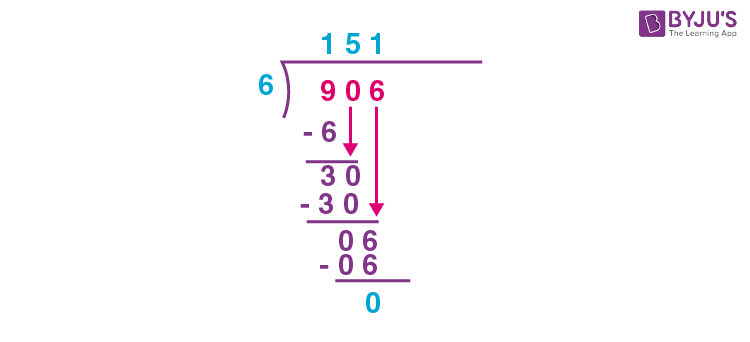Here, remainder = 0

Quotient = 151

2) Complete the division: 8503 ÷ 18 = ______ + 7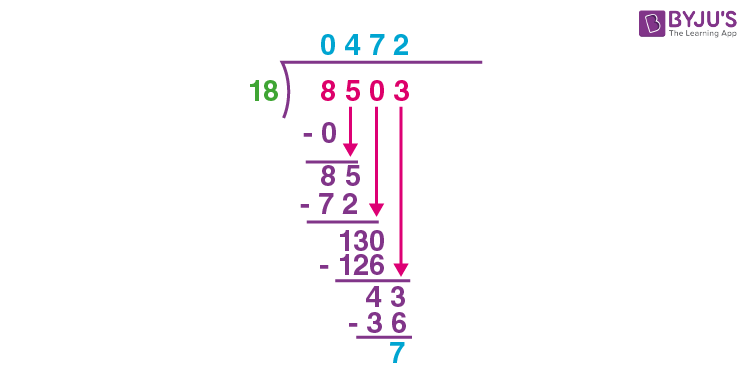Therefore, 8503 ÷ 18 = 18 × 472 + 7

3) Write the quotient when 2037 is divided by 12.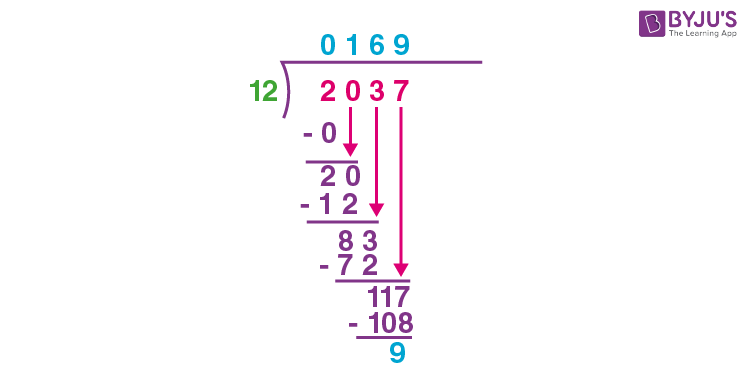Therefore, the quotient is 169.

### Division 3 Digits

In this section, you will learn how to divide a 3 digit number and division of numbers by a 3 digit number.

1) Perform division for 483 with 7 as a divisor.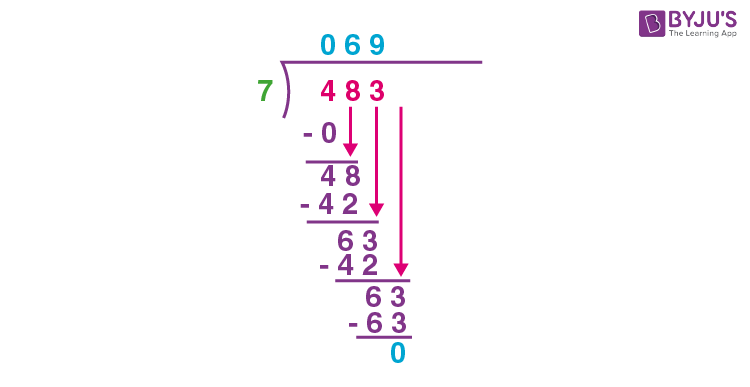Now, let’s have a look at the division of a number by a three-digit number.

2) Compute: 580654 ÷ 112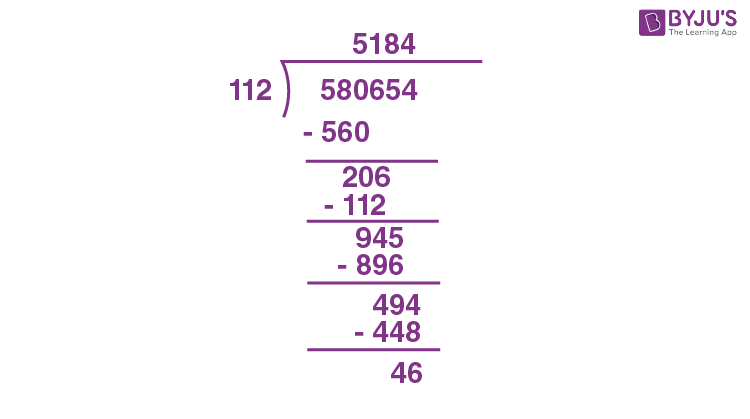### Division of Fractions

The division of fractions is quite different from the division of natural numbers. While dividing fractions, we have to convert the division operator to multiplication. That means the inverted divisor should be multiplied with the dividend. Let’s have a look at the below example.

Divide 12/5 by 16/3:

Numerator = 12/5

Denominator = 16/3

We can write the division of 12/5 by 16/3 as:

(12/5) ÷ (16/3)

= (12/5)/ (16/3)

Now, converting “÷” into “×” means that the divisor should be inverted and multiplied.

(12/5) × (3/16)

= (3/5) × (3/4)

= 9/20

Thus, the division 12/5 by 16/3 gives 9/20 as a result in fraction form.

## Division Examples

Below are examples of division problems in maths.

Example 1:

What is the remainder when 6585 is divided by 13?

Solution:

From the given,

Dividend = 6585

Divisor = 13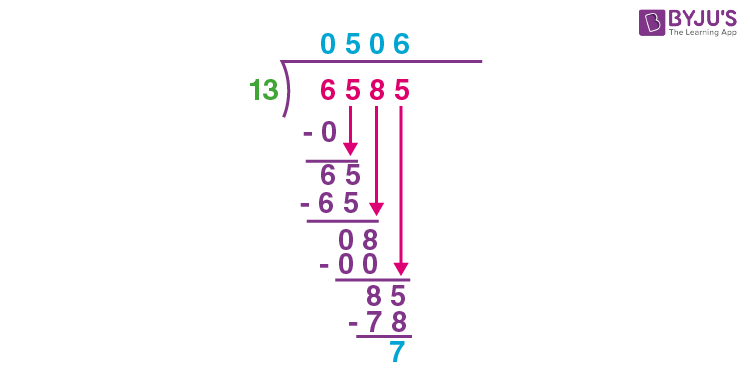Therefore, the remainder is equal to 7.

Example 2:

Divide 1764 by 11.

Solution:

From the given,

Dividend = 1764

Divisor = 11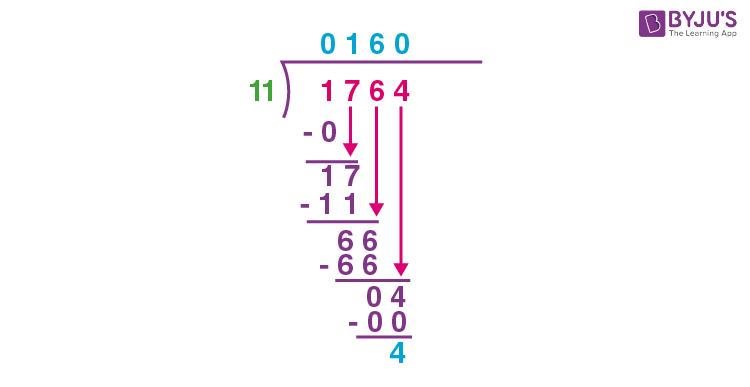Therefore, the division of 1764 by 11 gives the quotient as 160 by leaving the remainder 4.

### Practice Problems

1. Find the quotient when 6556 is divided by 4.
2. Divide 18187 by 137 and write the quotient and remainders.
3. What is the remainder when 17080 is divided by 54?

## Frequently Asked Questions on Division

### What is division in maths?

In maths, a division is a process of splitting a specific amount into equal parts. For example, we can divide a group of 20 members into 4 groups of 5 members each or 5 groups of 4 members each, and so on.

### What is the division algorithm formula?

The division algorithm formula is given as:
Dividend = Divisor × Quotient + Remainder

### What is the remainder formula?

The formula of the remainder is:
Remainder = Dividend – (Divisor × Quotient)

### What is the value of division by 0?

The division of any number by 0 gives infinity, i.e. the value of division by 0 is undefined.

### What is the symbol of division?

The symbols used to denote the division are ÷, slash (/) or a horizontal line ( _ ).